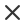FilterSort by
Media Type
Orientation
Number of people
Age
Ethnicity
Style
ColorMax 3 colors
Do not include these words

# Free Scientific Calculator Stock Photos And Images

Liquid flask and test tube neon light icon. organic chemistry. conducting experiment. mixing chemicals. scientific research. glowing sign with alphabet, symbols. vector isolated illustrationPLUSChemical reaction in lab flask neon light icon. organic chemistry. conducting experiment. laboratory work. interaction with chemicals. glowing sign with alphabet, symbols. vector isolated illustrationPLUSInfection test neon light icon. medical procedure. blood culture test. microscope with sample. hematology, microbiology. glowing sign with alphabet, numbers and symbols. vector isolated illustrationPLUSChemical experiment neon light icon. laboratory test tube with drop. glowing sign with alphabet, numbers and symbols. vector isolated illustrationPLUSChemical physics neon light icon. laboratory research. chemical substance in flask. lab experiment. biochemical reaction. glowing sign with alphabet, numbers and symbols. vector isolated illustrationPLUSStem education simple frame with black background - vector science technology engineering math concept outline illustrationPLUSTheory of relativity vector element can be used for theory, relativity, formula design concept. isolated formula flat icon.PLUSScientific calculator white icons in round glossy buttons with steel frames on black background. the buttons are in two different styles and eight colors.PLUSPapers, glasses and calculator to make calculations of the invoices we receive, to make graphs and statistics of our expenses or benefits. scientific calculator used by science studentsPLUS
Papers, glasses and calculator to make calculations of the invoices we receive, to make graphs and statistics of our expenses or benefits. scientific calculator used by science studentsPLUS
Mathematics, algebra and geometry subjects in school vector. economics university college discipline with finance calculation precision. charts infoPLUSPapers, glasses and calculator to make calculations of the invoices we receive, to make graphs and statistics of our expenses or benefits. scientific calculator used by science studentsPLUS
Testing reactives in lab flask neon light icon. organic chemistry. conducting experiment. laboratory work. interaction with chemicals. glowing sign with alphabet, symbols. vector isolated illustrationPLUSPapers, glasses and calculator to make calculations of the invoices we receive, to make graphs and statistics of our expenses or benefits. scientific calculator used by science studentsPLUS
Papers, glasses and calculator to make calculations of the invoices we receive, to make graphs and statistics of our expenses or benefits. scientific calculator used by science studentsPLUS
Papers, glasses and calculator to make calculations of the invoices we receive, to make graphs and statistics of our expenses or benefits. scientific calculator used by science studentsPLUS
Papers, glasses and calculator to make calculations of the invoices we receive, to make graphs and statistics of our expenses or benefits. scientific calculator used by science studentsPLUS
Calculator icon in flat style. calculate vector illustration on white isolated background. calculation business concept.PLUSScience, technology, engineering and math square frame with black background. vector stem concept outline illustrationPLUSPapers, glasses and calculator to make calculations of the invoices we receive, to make graphs and statistics of our expenses or benefits. scientific calculator used by science studentsPLUS
Science study vector icons set, modern solid symbol collection, filled style pictogram pack. signs, logo illustration. set includes icons as chemical research, geometric cube, genetic engineeringPLUSPapers, glasses and calculator to make calculations of the invoices we receive, to make graphs and statistics of our expenses or benefits. scientific calculator used by science studentsPLUS
Papers, glasses and calculator to make calculations of the invoices we receive, to make graphs and statistics of our expenses or benefits. scientific calculator used by science studentsPLUS
Papers, glasses and calculator to make calculations of the invoices we receive, to make graphs and statistics of our expenses or benefits. scientific calculator used by science studentsPLUS
Papers, glasses and calculator to make calculations of the invoices we receive, to make graphs and statistics of our expenses or benefits. scientific calculator used by science studentsPLUS
Papers, glasses and calculator to make calculations of the invoices we receive, to make graphs and statistics of our expenses or benefits. scientific calculator used by science studentsPLUS
Papers, glasses and calculator to make calculations of the invoices we receive, to make graphs and statistics of our expenses or benefits. scientific calculator used by science studentsPLUS
Papers, glasses and calculator to make calculations of the invoices we receive, to make graphs and statistics of our expenses or benefits. scientific calculator used by science studentsPLUS
Papers, glasses and calculator to make calculations of the invoices we receive, to make graphs and statistics of our expenses or benefits. scientific calculator used by science studentsPLUS
Papers, glasses and calculator to make calculations of the invoices we receive, to make graphs and statistics of our expenses or benefits. scientific calculator used by science studentsPLUS
Line style icon set design, science chemistry laboratory research technology biology equipment and test theme vector illustrationPLUSPapers, glasses and calculator to make calculations of the invoices we receive, to make graphs and statistics of our expenses or benefits. scientific calculator used by science studentsPLUS